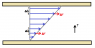# Fluid Mechanics shear stress, conceptual question

• zulfiqar6
The shear stress is the same.In summary, the shear stress in a fluid between two plates, one stationary and one moving at a constant velocity V, does not change as you move further from the moving plate. It is determined by the rate of change of velocity with respect to distance between the plates, which is constant throughout the region. This is due to the linear velocity profile between the plates, with a constant shear rate determined by the velocity and distance between the plates.

## Homework Statement

Consider a fluid between two plates. One on the bottom which is stationary, and one at the top which is moving at constant velocity V. The plates are separated by distance h.
Does shear stress change as you move further from the moving plate?

## Homework Equations

the equation given in the book is: τ = μ * (V/h)
there is another equation for velocity, u of the water at any height equals: (y/h) * V
where y is the y-coordinate, 0 at the bottom at V at the top, by the moving plate

## The Attempt at a Solution

where μ, V and h are constants. Therefore it seems shear force on the liquid is constant across the gap. but that doesn't make logical or intuitive sense to me. The further you get from the moving plate, the lower the velocity - doesn't that mean force on the liquid is smaller at greater depths?

Thanks.

Welcome to PF, zulfiqar6.

The shear force felt by a layer of water does not depend on the velocity of the layer with respect to the earth. It depends on the velocity of the layer relative to a neighboring layer. That is, it depends on the rate at which the velocity changes with respect to the distance y.

I still don't understand! Does shear stress change across the gap between the plates then, or not?

zulfiqar6 said:
I still don't understand! Does shear stress change across the gap between the plates then, or not?
It doesn't change. The shear stress in the fluid doesn't depend on the velocity. It depends on the rate of change of velocity with respect to distance between the plates. The velocity profile in the region between the plates is linear, from a value of 0 at the bottom plate to a value of V at the top plate. The velocity at any position y above the bottom plate is v = Vy/h, where h is the total distance between the plates. So, at any location between the plates, the rate of change of velocity with respect to distance y is the same: V/h. The shear stress is equal to this "shear rate" times the viscosity.

Here's a picture of the velocity profile between the plates. The blue vectors show how the velocity increases in the y direction. The stress depends on the "gradient" of the velocity, Δv/Δh, where Δv is the change in velocity when moving a small distance Δh in the y direction. In the picture you can see that Δv in going from layer 2 to 3 is the same as Δv in going from layer 6 to 7. The gradient of the velocity is the same near the bottom plate as it is near the top plate.

#### Attachments

•fluid stress.png
1.7 KB · Views: 467
Last edited: